Updating search results...

# 127 Results

View
Selected filters:
• Algebra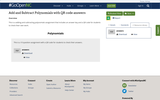Unrestricted Use
CC BY
Rating
0.0 stars
Overview:

This is a adding and subtracting polynomials assignment that includes an answer key and a QR code for students to check their own work.

Subject:
Algebra
Math 1
Material Type:
Homework/Assignment
Author:
KORI ELLIOTT
06/01/2020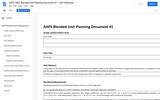Conditional Remix & Share Permitted
CC BY-NC
Rating
5.0 stars
Overview:

Students are expected to learn how to factor polynomials with an emphasis on quadratic expressions.

Subject:
Mathematics
Algebra
Material Type:
Unit of Study
Provider:
Michigan Virtual
Author:
Jeff Oleksinski
04/11/2017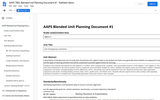Conditional Remix & Share Permitted
CC BY-NC-SA
Rating
3.0 stars
Overview:

Students have already had experience with patterns of numbers when studying linear, quadratic, and exponential functions in both Algebra 1 and Algebra 2. This is their first introduction to the formal notation used for sequences and summation notation for series. Students will explore series and summation notation. Arithmetic sequences and series, including finding common differences, terms, and partial sums will be examined in this unit. Students will also learn about geometric sequences and series, including finding common ratios, terms, partial sums, and infinite sums. Students will also explore how to write numbers with repeating decimals as fractions in simplest form using infinite geometric series and common ratio concepts.

Subject:
Mathematics
Algebra
Material Type:
Unit of Study
Provider:
Michigan Virtual
Author:
Kathleen Ilaoro
03/24/2017Rating
4.0 stars
Overview:

This is a visual demonstration of the sector-proof of the formula for the area of circle.

Subject:
Mathematics
Algebra
Math 1
Material Type:
Demonstration
Provider:
GeoGebra
Author:
GeoGebra
02/26/2019Conditional Remix & Share Permitted
CC BY-NC-SA
Rating
0.0 stars
Overview:

This task explores the real world topic of building light rails. Throughout the implementation of this task the students will learn about the cost of building railways and how to implement them within a budget. This task explores such mathematical concepts of using coordinates to find the distance between points, using coordinates to build polygons and find the area and length of sides, and writing equations of parallel lines.

Subject:
Algebra
Geometry
Material Type:
Activity/Lab
Author:
Carrie Robledo
James O'Neal
03/26/2021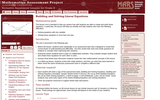Only Sharing Permitted
CC BY-NC-ND
Rating
4.0 stars
Overview:

This lesson unit is intended to help teachers assess how well students are able to create and solve linear equations. In particular, the lesson will help you identify and help students who have the following difficulties: solving equations with one variable and solving linear equations in more than one way.

Subject:
Algebra
Material Type:
Assessment
Lesson Plan
Provider:
Shell Center for Mathematical Education
Provider Set:
Mathematics Assessment Project (MAP)
06/24/2019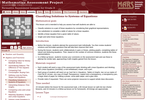Only Sharing Permitted
CC BY-NC-ND
Rating
4.0 stars
Overview:

This lesson unit is intended to help teachers assess how well students are able to classify solutions to a pair of linear equations by considering their graphical representations. In particular, this unit aims to help teachers identify and assist students who have difficulties in: using substitution to complete a table of values for a linear equation; identifying a linear equation from a given table of values; and graphing and solving linear equations.

Subject:
Algebra
Material Type:
Assessment
Lesson Plan
Provider:
Shell Center for Mathematical Education
Provider Set:
Mathematics Assessment Project (MAP)
06/24/2019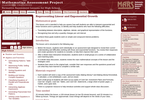Only Sharing Permitted
CC BY-NC-ND
Rating
4.0 stars
Overview:

This lesson unit is intended to help teachers assess how well students are able to interpret exponential and linear functions and in particular to identify and help students who have the following difficulties: translating between descriptive, algebraic and tabular data, and graphical representation of the functions; recognizing how, and why, a quantity changes per unit intervale; and to achieve these goals students work on simple and compound interest problems.

Subject:
Algebra
Geometry
Material Type:
Assessment
Lesson Plan
Provider:
Shell Center for Mathematical Education
Provider Set:
Mathematics Assessment Project (MAP)
06/24/2019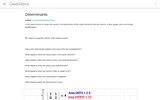Conditional Remix & Share Permitted
CC BY-SA
Rating
0.0 stars
Overview:

This dynamic worksheet illustrates the relationship between the DETERMINANT, the Area of the pre-image, and the Area of the image.

Subject:
Algebra
Material Type:
Simulation
Provider:
GeoGebra
Provider Set:
GeoGebraTube
04/26/2012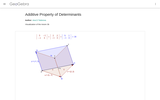Conditional Remix & Share Permitted
CC BY-SA
Rating
0.0 stars
Overview:

Ana Donevska Todorova Humboldt Universitﾊt zu Berlin Mathematisch-Naturwissenschaftliche Fakultﾊt II Institut fﾟr Mathematik, Didaktik der Mathematik ??? ?????????? ?????? ???????? ?? ???????????? ????? ? ????

Subject:
Algebra
Material Type:
Simulation
Provider:
GeoGebra
Provider Set:
GeoGebraTube
03/12/2012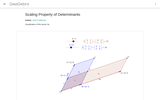Conditional Remix & Share Permitted
CC BY-SA
Rating
0.0 stars
Overview:

Ana Donevska Todorova Humboldt Universitﾊt zu Berlin Mathematisch-Naturwissenschaftliche Fakultﾊt II Institut fﾟr Mathematik, Didaktik der Mathematik

Subject:
Algebra
Material Type:
Simulation
Provider:
GeoGebra
Provider Set:
GeoGebraTube
03/12/2012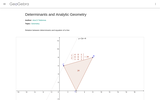Conditional Remix & Share Permitted
CC BY-SA
Rating
0.0 stars
Overview:

Ana Donevska Todorova Humboldt Universitﾊt zu Berlin Mathematisch-Naturwissenschaftliche Fakultﾊt II Institut fﾟr Mathematik, Didaktik der Mathematik

Subject:
Algebra
Material Type:
Simulation
Provider:
GeoGebra
Provider Set:
GeoGebraTube
03/12/2012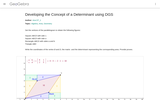Conditional Remix & Share Permitted
CC BY-SA
Rating
0.0 stars
Overview:

Developing the Concept of a Determinant using DGS

Subject:
Algebra
Geometry
Material Type:
Simulation
Provider:
GeoGebra
Provider Set:
GeoGebraTube
11/11/2011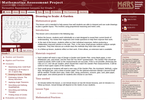Only Sharing Permitted
CC BY-NC-ND
Rating
3.5 stars
Overview:

This lesson unit is intended to help assess how well students are able to interpret and use scale drawings to plan a garden layout. This involves using proportional reasoning and metric units.

Subject:
Algebra
Geometry
Material Type:
Assessment
Lesson Plan
Provider:
Shell Center for Mathematical Education
Provider Set:
Mathematics Assessment Project (MAP)
06/24/2019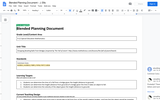Conditional Remix & Share Permitted
CC BY-NC-SA
Rating
4.0 stars
Overview:

Calculating time, distance, and speed of falling objects.

Subject:
Mathematics
Algebra
Material Type:
Activity/Lab
Provider:
Michigan Virtual
Author:
Jonny Ellis
06/14/2017Unrestricted Use
Public Domain
Rating
0.0 stars
Overview:

EXERCISING IN SPACE

Algebra 1

Key Topic: Slope, linear equations, direct variation, independent and dependent variables, different representations of a function

Students will identify a direct variation, solve linear equations, and create tables based on the exercise equipment provided for astronauts on long duration missions on the International Space Station (ISS) in order to maintain their fitness to perform mission objectives and to return to Earth without serious health complications.

Students will
identify direct variation from ordered pairs by calculating the constant of variation;
calculate slope from two points using the slope formula;
determine independent and dependent variables;
solve linear equations; and
create tables.

Prerequisites: Students should have prior knowledge of direct variation, the constant of variation, linear functions, different representations of a linear function, the properties of a linear function, calculating slope, and solving linear equations.

Subject:
Algebra
Material Type:
Lesson
Author:
NASA
07/22/2020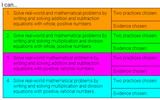Conditional Remix & Share Permitted
CC BY-NC-SA
Rating
0.0 stars
Overview:

Students will build their learning on one-step equations. Students will be able to choose what they complete for each section which gives them some variability in their learning. Students are also able to choose between taking a typical test or completing a "more fun" activity such as creating a poster or videoing themself teaching a couple problems. This resource was developed as part of a professional learning opportunity funded by the NCDPI Digital Learning Initiative Planning Grant/

Subject:
Mathematics
Algebra
Material Type:
Activity/Lab
Assessment
Homework/Assignment
Vocabulary
Author:
JORDAN KING
06/04/2021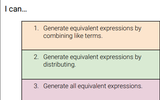Conditional Remix & Share Permitted
CC BY-NC-SA
Rating
0.0 stars
Overview:

Students will build their learning on combining like terms, distributive property, and combining like terms when using the distributive property. Students will be able to choose what they complete for each section which gives them some variability in their learning. Students are also able to choose between taking a typical test or completing a "more fun" activity such as creating a poster or videoing themself teaching a couple problems. This resource was developed as part of a professional learning opportunity funded by the NCDPI Digital Learning Initiative Planning Grant.

Subject:
Algebra
Material Type:
Activity/Lab
Assessment
Formative Assessment
Lesson Plan
Vocabulary
Author:
JORDAN KING
06/04/2021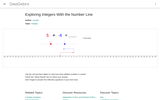Conditional Remix & Share Permitted
CC BY-NC-SA
Rating
4.0 stars
Overview:

This simulation allows you to use a slider to see how positive and negative integers add on a number line.

Subject:
Mathematics
Algebra
Material Type:
Diagram/Illustration
Simulation
Provider:
GeoGebra
Author:
rsnyder
07/31/2019Rating
0.0 stars
Overview:

A Constructed Response Item provided by Smarter Balanced as preliminary examples of the types of items that students might encounter on the summative assessment. The item prompt students to produce a text or numerical response in order to collect evidence about their knowledge or understanding of writing a function defined by an expression in different but equivalent forms to reveal and explain different properties of a LINEAR function. MAT.HS.CR.1.00FIF.M.274

Subject:
Mathematics
Algebra
Math 1
Material Type:
Activity/Lab
Assessment
Provider:
Oregon Department of Education
Author:
Smarter Balanced Assessment Consortium
02/26/2019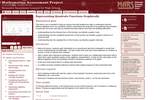Only Sharing Permitted
CC BY-NC-ND
Rating
4.0 stars
Overview:

This lesson unit is intended to help teachers assess how well students are able to understand what the different algebraic forms of a quadratic function reveal about the properties of its graphical representation. In particular, the lesson will help teachers identify and help students who have the following difficulties: understanding how the factored form of the function can identify a graphŐs roots; understanding how the completed square form of the function can identify a graphŐs maximum or minimum point; and understanding how the standard form of the function can identify a graphŐs intercept.

Subject:
Algebra
Material Type:
Assessment
Lesson Plan
Provider:
Shell Center for Mathematical Education
Provider Set:
Mathematics Assessment Project (MAP)
06/24/2019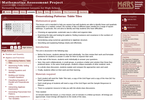Only Sharing Permitted
CC BY-NC-ND
Rating
5.0 stars
Overview:

This lesson unit is intended to help teachers assess how well students are able to identify linear and quadratic relationships in a realistic context: the number of tiles of different types that are needed for a range of square tabletops. In particular, this unit aims to identify and help students who have difficulties with: choosing an appropriate, systematic way to collect and organize data; examining the data and looking for patterns; finding invariance and covariance in the numbers of different types of tile; generalizing using numerical, geometrical or algebraic structure; and describing and explaining findings clearly and effectively.

Subject:
Algebra
Geometry
Material Type:
Assessment
Lesson Plan
Provider:
Shell Center for Mathematical Education
Provider Set:
Mathematics Assessment Project (MAP)
07/31/2019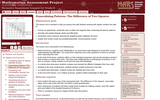Only Sharing Permitted
CC BY-NC-ND
Rating
5.0 stars
Overview:

This lesson unit is intended to help you assess how well students working with square numbers are able to: choose an appropriate, systematic way to collect and organize data, examining the data for patterns; describe and explain findings clearly and effectively; generalize using numerical, geometrical, graphical and/or algebraic structure; and explain why certain results are possible/impossible, moving towards a proof.

Subject:
Algebra
Geometry
Material Type:
Assessment
Lesson Plan
Provider:
Shell Center for Mathematical Education
Provider Set:
Mathematics Assessment Project (MAP)
07/31/2019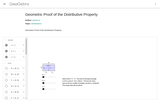Conditional Remix & Share Permitted
CC BY-NC-SA
Rating
0.0 stars
Overview:

This resource demonstrates the distributive property through the area of rectangles. You can manipulate the dimension by moving the slider.

Subject:
Mathematics
Algebra
Geometry
Material Type:
Diagram/Illustration
Simulation
Provider:
GeoGebra
Author:
jtmace11
07/31/2019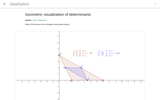Conditional Remix & Share Permitted
CC BY-SA
Rating
0.0 stars
Overview:

Ana Donevska Todorova Humboldt Universitﾊt zu Berlin Mathematisch-Naturwissenschaftliche Fakultﾊt II Institut fﾟr Mathematik, Didaktik der Mathematik

Subject:
Algebra
Material Type:
Simulation
Provider:
GeoGebra
Provider Set:
GeoGebraTube
03/12/2012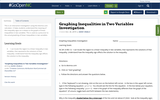Conditional Remix & Share Permitted
CC BY-NC-SA
Rating
0.0 stars
Overview:

This is an interactive investigation using the Desmos.com calculator. It helps students understand the meaning of the inequality signs as it relates to the graphs of linear inequalities in two variables. This is used as a precursor to the actual graphing of linear inequalities in two variables.

Subject:
Algebra
Material Type:
Activity/Lab
Formative Assessment
Interactive
Author:
MARIE VRABLIC
11/06/2019Only Sharing Permitted
CC BY-NC-ND
Rating
0.0 stars
Overview:

Students will use a video about two rival competitive eaters to explore linear and quadratic patterns.

Subject:
Algebra
Math 1
Material Type:
Activity/Lab
Formative Assessment
Author:
Robert Kaplinsky
11/26/2019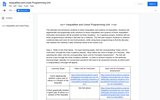Conditional Remix & Share Permitted
CC BY-NC-SA
Rating
3.0 stars
Overview:

This blended unit introduces students to linear inequalities and systems of inequalities. Students will algebraically and graphically verify solutions to linear inequalities and systems of linear inequalities.  Instruction and practice will be provided via Khan Academy.  As a capstone project, students will use linear programming to develop a diet plan for a celebrity; the diet plan requires students to research nutritional data and costs for two food products, while using linear programming to find the diet plan that satisfies the celebrity's daily nutritional needs at the lowest cost.

Subject:
Mathematics
Algebra
Material Type:
Unit of Study
Provider:
Michigan Virtual
Author:
Raymond Herek
06/13/2017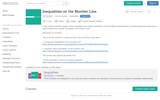Only Sharing Permitted
CC BY-NC-ND
Rating
0.0 stars
Overview:

This lesson module allows students to explore the meaning of inequalities. After this lesson, students should be able to understand that there are multiple solutions to an inequality. It contains a formative assessment matching activity at the end and a critical thinking closing activity.

Subject:
Algebra
Math 1
Material Type:
Activity/Lab
Formative Assessment
Vocabulary
Author:
Desmos.com
11/27/2019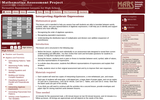Only Sharing Permitted
CC BY-NC-ND
Rating
4.0 stars
Overview:

This lesson unit is intended to help teachers assess how well students are able to translate between words, symbols, tables, and area representations of algebraic expressions. It will help teachers to identify and support students who have difficulty in: recognizing the order of algebraic operations; recognizing equivalent expressions; and understanding the distributive laws of multiplication and division over addition (expansion of parentheses).

Subject:
Algebra
Material Type:
Assessment
Lesson Plan
Provider:
Shell Center for Mathematical Education
Provider Set:
Mathematics Assessment Project (MAP)
06/24/2019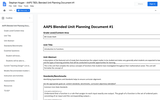Conditional Remix & Share Permitted
CC BY-SA
Rating
4.0 stars
Overview:

This is a unit that formalizes the definition of functions. Students will connect the idea of mathematical relationships to the concept of functions. With a mix of direct instruction, guided notes with procedural practice, and individual practice, this unit will ground students in the concept and provide a deep understanding of its usefulness.

Subject:
Mathematics
Algebra
Material Type:
Unit of Study
Provider:
Michigan Virtual
Author:
Stephan Hogan
09/19/2017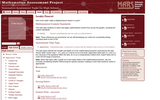Rating
0.0 stars
Overview:

Students must determine how much water might a dripping faucet waste in a year. This task asks students to select and apply mathematical content from across the grades, including the content standards.

Subject:
Mathematics
Algebra
Math 2
Material Type:
Activity/Lab
Assessment
Formative Assessment
Provider:
MARS, Mathematics Assessment Resource Service
Author:
Shell Center Team
06/24/2019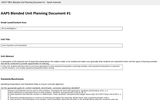Unrestricted Use
CC BY
Rating
5.0 stars
Overview:

In this unit, students will learn how to use inverse operations to solve equations/inequalities containing variables, write equations/inequalities to represent real life situations, and simplify equations/inequalities before solving.

Subject:
Mathematics
Algebra
Material Type:
Unit of Study
Provider:
Michigan Virtual
Author:
Sarah Azarovitz
09/11/2016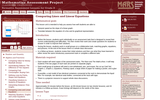Only Sharing Permitted
CC BY-NC-ND
Rating
4.0 stars
Overview:

This lesson unit is intended to help teahcers assess how well students are able to interpret speed as the slope of a linear graph and translate between the equation of a line and its graphical representation.

Subject:
Algebra
Material Type:
Assessment
Lesson Plan
Provider:
Shell Center for Mathematical Education
Provider Set:
Mathematics Assessment Project (MAP)
06/24/2019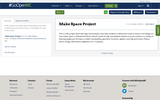Conditional Remix & Share Permitted
CC BY-NC
Rating
0.0 stars
Overview:

A PBL project that allows high school physics and math students exercise voice & choice in the design of a new maker space.

Subject:
Engineering
Algebra
Geometry
Math 2
Trigonometry
Physics
Material Type:
Unit of Study
Author:
Ben Owens
11/06/2019Rating
0.0 stars
Overview:

Equations and Inequalities

Type of Unit: Concept

Prior Knowledge

Students should be able to:

Add, subtract, multiply, and divide with whole numbers, fractions, and decimals.
Use the symbols <, >, and =.
Evaluate expressions for specific values of their variables.
Identify when two expressions are equivalent.
Simplify expressions using the distributive property and by combining like terms.
Use ratio and rate reasoning to solve real-world problems.
Order rational numbers.
Represent rational numbers on a number line.

Lesson Flow

In the exploratory lesson, students use a balance scale to find a counterfeit coin that weighs less than the genuine coins. Then continuing with a balance scale, students write mathematical equations and inequalities, identify numbers that are, or are not, solutions to an equation or an inequality, and learn how to use the addition and multiplication properties of equality to solve equations. Students then learn how to use equations to solve word problems, including word problems that can be solved by writing a proportion. Finally, students connect inequalities and their graphs to real-world situations.

Subject:
Mathematics
Algebra
Provider:
Pearson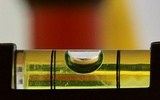Conditional Remix & Share Permitted
CC BY-NC
Rating
0.0 stars
Overview:

Subject:
Algebra
Material Type:
Lesson Plan
Author:
Pearson
11/02/2020Conditional Remix & Share Permitted
CC BY-NC
Rating
0.0 stars
Overview:

Lesson OverviewStudents apply the multiplication property of equality to solve equations.Key ConceptsIn the previous lesson, students solved equations of the form x + p = q using the addition property of equality. In this lesson, they will solve equations of the form px = q using the multiplication property of equality. They will multiply or divide both sides of an equation by the same number to obtain an equivalent equation.Since multiplication by a is equivalent to division by 1a, students will see that they may also divide both sides of the equation by the same number to get an equivalent equation. Students will also apply this property to solving a particular kind of equation, a proportion.Goals and Learning ObjectivesUse the multiplication property of equality to keep an equation balanced.Use the multiplication property of equality to solve equations of the form px = q for cases in which p, q, and x are all non-negative rational numbers.Use the multiplication property of equality to solve proportions.

Subject:
Algebra
Material Type:
Lesson Plan
Author:
Pearson
11/02/2020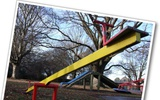Conditional Remix & Share Permitted
CC BY-NC
Rating
0.0 stars
Overview:

Gallery OverviewAllow students who have a clear understanding of the content thus far in the unit to work on Gallery problems of their choosing. You can then use this time to provide additional help to students who need review of the unit's concepts or to assist students who may have fallen behind on work.Gallery DescriptionsKeep It BalancedStudents will use reasoning to complete some equations to make them true.Equation SortStudents will sort equations into three groups: equations with one solution, equations with many solutions, and equations with no solutionsOn the Number LineStudents will use a number line to identify numbers that make an equation or inequality true.How Many Colors?Students will write and solve an equation to find the number of different colored blocks in a box.Value of sStudents will use a property of equality to solve an equation with large numbers.Marbles in a CupStudents are given information about the weight of a cup with two different amounts of marbles in it. They use this information to find the weight of the cup.When Is It True?Students will use what they know about 0 and 1 to decide when a certain equation is true.

Subject:
Algebra
Material Type:
Lesson Plan
Author:
Pearson
11/02/2020Conditional Remix & Share Permitted
CC BY-NC
Rating
0.0 stars
Overview:

Lesson OverviewStudents practice solving equations using either the addition or the multiplication property of equality.Key ConceptsStudents will solve equations of the form x + p = q using the addition property of equality.They will solve equations of the form px = q using the multiplication property of equality.They will need to look at the variable and decide what operation must be performed on both sides of the equation in order to isolate the variable on one side of the equation.If a number has been added to the variable, they will subtract that number from both sides of the equation. If a number has been subtracted from the variable, they will add that number to both sides of the equation. If the variable has been multiplied by a number, students will either divide both sides of the equation by that number or multiply by the reciprocal of that number. If the variable has been divided by a number, students will multiply by that number. Students will see how this can be applied to solving a proportion such as xc=ab.Goals and Learning ObjectivesPractice solving equations using either the addition or the multiplication property of equality.Distinguish between equations that can be solved using the addition property of equality from equations that can be solved using the multiplication property of equality.Solve a proportion by solving an equation.

Subject:
Algebra
Material Type:
Lesson Plan
Author:
Pearson